Latest Banking jobs   »   NIACL AO 2021 Result Out, Mains Exam Date For 300 AO Recruitment   »   Quantitative Aptitude Quiz For IBPS Clerk/NIACL...

# Quantitative Aptitude Quiz For IBPS Clerk/NIACL AO Prelims 2021- 21st September

Directions (1–5): In each of these questions, two equations (I) and (II) are given. You have to solve both the equations and answer the following questions.
(a) if x>y
(b) if x≥y
(c) if x<y
(d) if x ≤y
(e) if x = y or no relation can be established between x and y.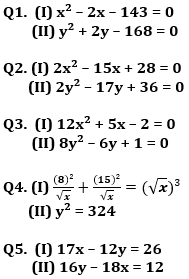Directions (6-10): Solve the given quadratic equations and mark the correct option based on your answer—
(a) x > y
(b) x ≥ y
(c) x < y
(d) x ≤ y
(e) x = y or no relation can be established between x and y.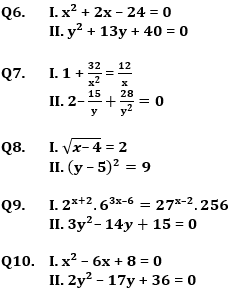Directions (11-15): In each of these questions, two equations (I) and (II) are given. You have to solve both the equations and give answer
(a) if x>y
(b) if x≥y
(c) if x<y
(d) if x ≤y
(e) if x = y or no relation can be established between x and y.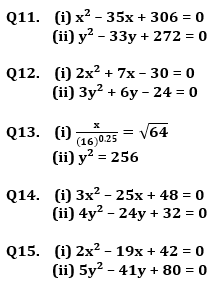Solutions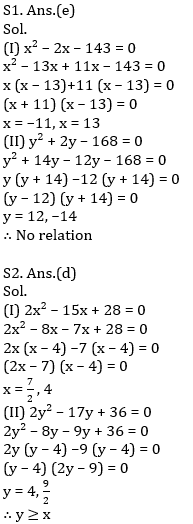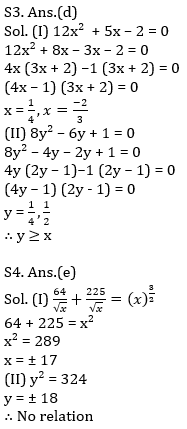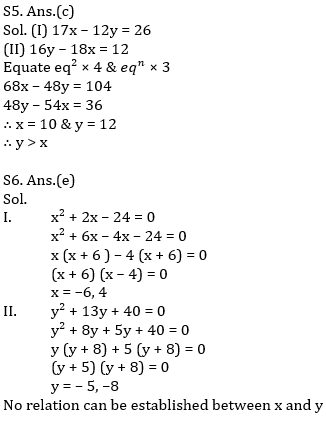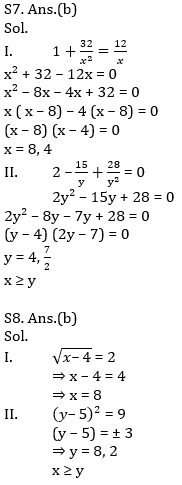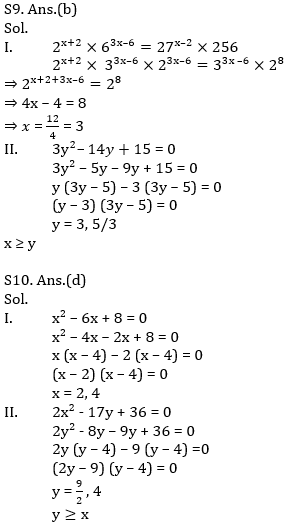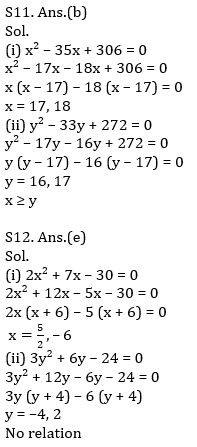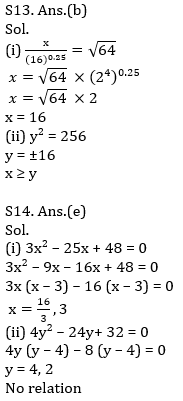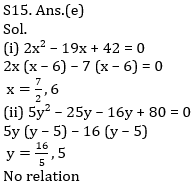Click Here to Register for Bank Exams 2021 Preparation Material#### Congratulations!Download Hindu Review of October 2021: Free PDF